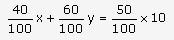# If in a rectangle, the length is increased and breadth is reduced each

If in a rectangle, the length is increased and breadth is reduced each by 2 metres, the area is reduced by 28 sq mtrs. If the length is reduced by 1 metre and breadth is increased by 2 metres, the area is increased by 33 sq mtrs. Find the length and breadth of the rectangle.
or
A chemist has one solution which is 40% acid and a second which is 60% acid. How much of each should be mixed to make 10 litres of 50% acid solution.So the length and breadth of the rectangle are 23 metres and 11 metres respectively.
OR
Let 40 % acids in the solution be x litres
Let 60 % of other solution be y litres
Total Volume in the mixture = x + y
Given volume is 10 litres
x + y = 10 —(i)
Also,So, 40 x + 60 y =500 or 2x+3y =25 …(ii)
Solving (i) and (ii) we get x = y = 5 litres